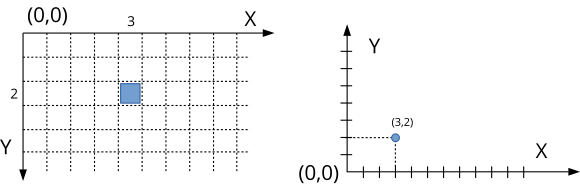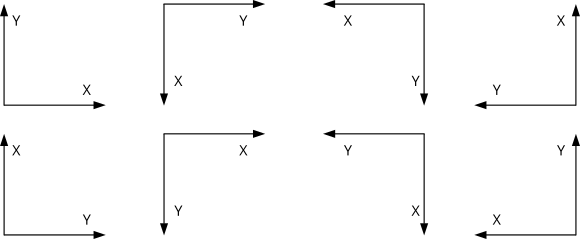# Geom2D

This page has been automatically translated using the Google Translate API services. We are working on improving texts. Thank you for your understanding and patience.

## Types and Constants

 struct V2D struct S2D struct R2D struct T2D struct Seg2D struct Cir2D struct Box2D struct OBB2D struct Tri2D struct Pol2D struct Col2D

We are facing a geometric calculation library in two dimensions. Geom2D allows working with primitives in the real plane: Points, vectors, transformations, curves and surfaces. It offers only mathematical functionality, that is, it does not define any type of representation or drawing operation. It only depends on Core library (Figure 1), so it can be used in both desktop applications and command line utilities. All types and functions are defined in simple `(float)` and `double` precision, in addition to being able to make use of C++ Math templates.

All geometric elements are based on (x, y) coordinates in the plane. Geom2D does not assume how these coordinates will be interpreted. That will depend on the reference system defined by the application. The most used are the Cartesian and the screen (Figure 2), although others systems could be used where appropriate (Figure 3).Figure 2: Interpretation of the coordinate (3,2) on monitors (left) and on the Cartesian plane (right).Figure 3: Different 2D coordinate systems.

## struct V2D

Represents a 2d vector or point. 2D Vectors.

```struct V2Df
{
real32_t x;
real32_t y;
};

struct V2Dd
{
real64_t x;
real64_t y;
};

template<typename real>
struct V2D
{
real x;
real y;
};
```
 x Coordinate x. y Coordinate y.

## struct S2D

Represents a 2d size. 2D Size.

```struct S2Df
{
real32_t width;
real32_t height;
};

struct S2Dd
{
real64_t width;
real64_t height;
};

template<typename real>
struct S2D
{
real width;
real height;
};
```
 width Width. height Height.

## struct R2D

2d rectangle. 2D Rectangles.

```struct R2Df
{
V2Df pos;
S2Df size;
};

struct R2Dd
{
V2Dd pos;
S2Dd size;
};

template<typename real>
struct R2D
{
V2D pos;
S2D size;
};
```
 pos Origin. size Size.

## struct T2D

2d affine transformation. 2D Transformations.

```struct T2Df
{
V2Df i;
V2Df j;
V2Df p;
};

struct T2Dd
{
V2Dd i;
V2Dd j;
V2Dd p;
};

template<typename real>
struct T2D
{
V2D i;
V2D j;
V2D p;
};
```
 i Component i of the linear transformation. j Component j of the linear transformation. p Position.

## struct Seg2D

2d line segment. 2D Segments.

```struct Seg2Df
{
V2Df p0;
V2Df p1;
};

struct Seg2Dd
{
V2Dd p0;
V2Dd p1;
};

template<typename real>
struct Seg2D
{
V2D p0;
V2D p1;
};
```
 p0 Coordinate of the first point of the segment. p1 Coordinate of the second point of the segment.

## struct Cir2D

2d circle. 2D Circles.

```struct Cir2Df
{
V2Df c;
real32_t r;
};

struct Cir2Dd
{
V2Dd c;
real64_t r;
};

template<typename real>
struct Cir2D
{
V2D c;
real r;
};
```

## struct Box2D

2d bounding box. 2D Boxes.

```struct Box2Df
{
V2Df min;
V2Df max;
};

struct Box2Dd
{
V2Dd min;
V2Dd max;
};

template<typename real>
struct Box2D
{
V2D min;
V2D max;
};
```
 min Minimum bounding coordinate. max Maximum bounding coordinate.

## struct OBB2D

2d Oriented Bounding Box. 2D Oriented Boxes.

```struct OBB2Df;

struct OBB2Dd;

template<typename real>
struct OBB2D;
```

## struct Tri2D

2d triangle. 2D Triangles.

```struct Tri2Df
{
V2Df p0;
V2Df p1;
V2Df p2;
};

struct Tri2Dd
{
V2Dd p0;
V2Dd p1;
V2Dd p2;
};

template<typename real>
struct Tri2D
{
V2D p0;
V2D p1;
V2D p2;
};
```
 p0 Coordinate of the first point of the triangle. p1 Coordinate of the second point of the triangle. p2 Coordinate of the third point of the triangle.

## struct Pol2D

2d convex polygon. 2D Polygons.

```struct Pol2Df;

struct Pol2Dd;

template<typename real>
struct Pol2D;
```

## struct Col2D

Collision data in 2d. 2D Collisions.

```struct Col2Df;

struct Col2Dd;

template<typename real>
struct Col2D;
```